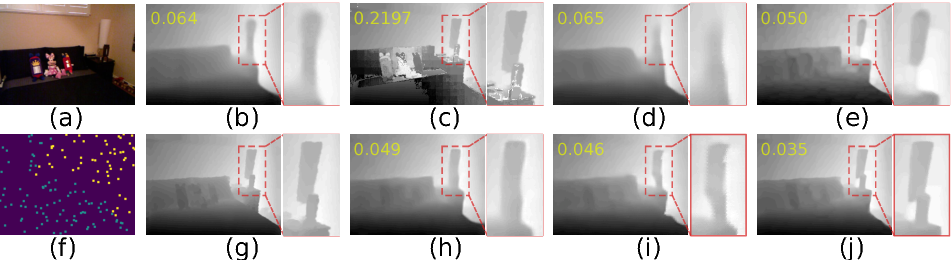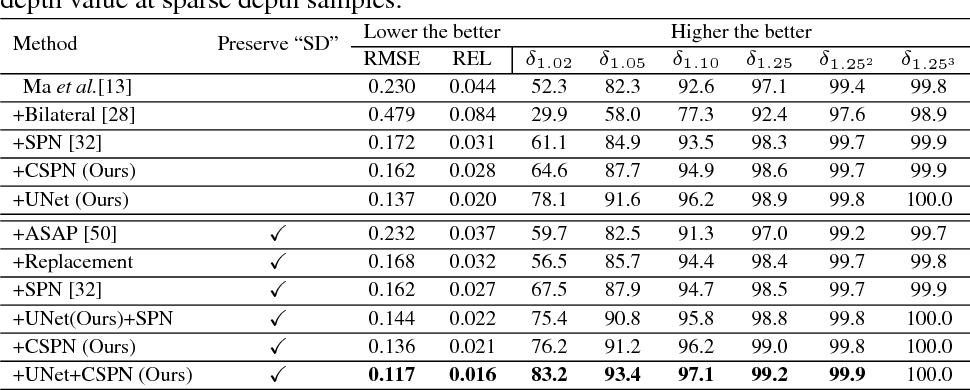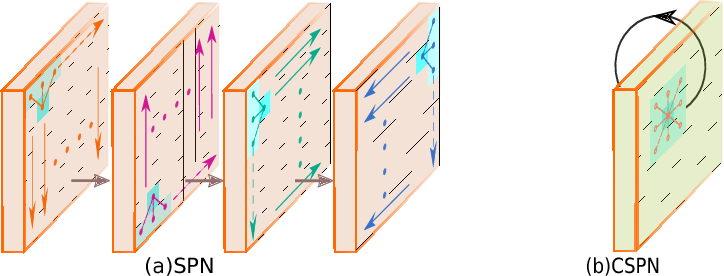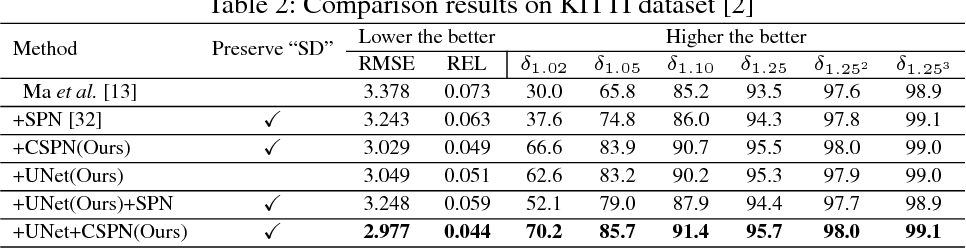Depth estimation from a single image is a fundamental problem in computer vision. In this paper, we propose a simple yet effective convolutional spatial propagation network (CSPN) to learn the affinity matrix for depth prediction. Specifically, we adopt an efficient linear propagation model, where the propagation is performed with a manner of recurrent convolutional operation, and the affinity among neighboring pixels is learned through a deep convolutional neural network (CNN). We apply the designed CSPN to two depth estimation tasks given a single image: (1) To refine the depth output from state-of-the-art (SOTA) existing methods; and (2) to convert sparse depth samples to a dense depth map by embedding the depth samples within the propagation procedure. The second task is inspired by the availability of LIDARs that provides sparse but accurate depth measurements. We experimented the proposed CSPN over two popular benchmarks for depth estimation, i.e. NYU v2 and KITTI, where we show that our proposed approach improves in not only quality (e.g., 30% more reduction in depth error), but also speed (e.g., 2 to 5 times faster) than prior SOTA methods.

* 14 pages, 8 figures, ECCV 2018Click To Get Code For Paper